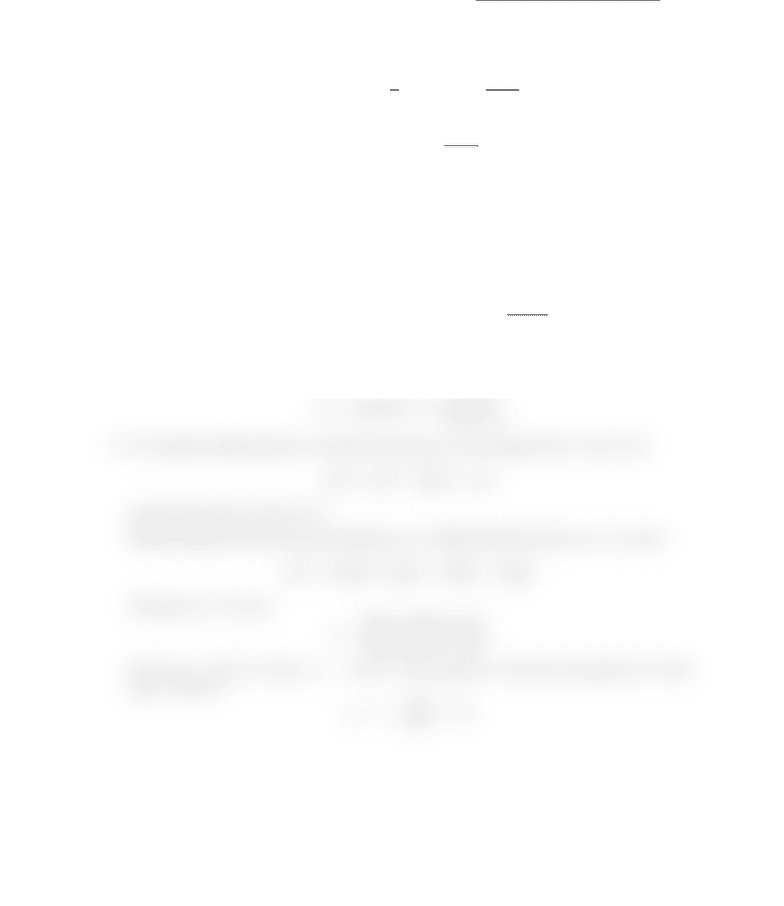Study Guides (380,000)
US (220,000)
CU-Boulder (1,000)
MATH (100)
All (40)
Quiz

# MATH 1300 Study Guide - Quiz Guide: Algebraic Function, Inverse Trigonometric FunctionsExam

Department
Mathematics
Course Code
MATH 1300
Professor
All
Study Guide
Quiz

This preview shows half of the first page. to view the full 2 pages of the document.Math 1300-001, Quiz 5 Name:
1. What are the derivatives of the following functions? (You should know all of these!)
(a) ln xand log3x
(ln x)0=1
x,(log3x)0=1
xln 3.
In general
(logax)0=1
xln a.
(b) exand 3x
(ex)0=ex,(3x)0= 3xln 3.
In general
(ax)0=axln a.
(c) tan xand arctan x
(tan x)0= sec2x, (arctan x)0=1
1 + x2.
2. Find the derivative of
y= sin(ln(3x2+ 2))
y0= cos(ln(3x2+ 2)) 6x
3x2+ 2.
3. Use implicit diﬀerentiation to ﬁnd the equation of the tangent line to the curve
2(x2+y2)2= 25(x2
y2)
going through the point (3,1).
Diﬀerentiating both sides and assuming yis a diﬀerentiable function of xwe have
4(x2+y2)(2x+ 2yy0) = 25(2x2yy0).
Solving for y0we have
y0=50x8x(x2+y2)
50y+ 8y(x2+y2).
At (x, y) = (3,1) we have y0=9/13. The equation of the line through (3,1) with
slope 9/13 is
y1 = 9
13 (x3).
###### You're Reading a Preview

Unlock to view full version